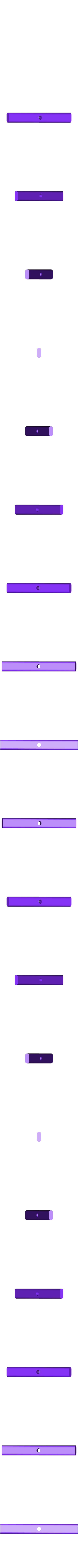# Parabola Manipulative## 3D model description

Use this manipulative to build an intuitive understanding of how the "b" coefficient of a quadratic function affects the graphed parabola.

Here's a quick overview video:

Given a quadratic equation of the form:

y = ax^2 + bx + c

The "a" coefficient stretches the parabola vertically. The "c" coefficient moves the parabola up and down. These are easy to understand. But the "b" coefficient is a little more tricky. Use this manipulative to get a feel for how it works.

For teachers I've included the following:

1) Non-verbal instructions for assembling (pdf file). I like giving students opportunities to practice following visual instructions.

2) Exploration Guide (pdf file). Good questions for project-based learners to explore parabolas using this manipulative.

3) A game-based virtual manipulative for students to interact with online:

Here are the Common Core standards connected to this manipulative: F_IF.7, G_GPE_3.1 (California addition), G_GPE.1 and G_GPE.2.

The source files in SketchUp are also provided in a zip archive.

## 3D printing settings

Print out all the parts and then follow the visual instructions in the Visual_Instructions.pdf file.

Here are the colored filaments I used for the different parts:

WHITE: Parabola Backbone.stl, Parabola Frame.stl, Parabola Slope Knob.stl

BLACK: Parabola Slope Bar.stl, Parabola Slope Pin.stl

BLUE: Parabola Rods.stl

• 3D model format: STL and ZIP